# Common Core: 6th Grade Math : Fluently Add, Subtract, Multiply, and Divide Multi-Digit Decimals: CCSS.Math.Content.6.NS.B.3

## Example Questions

← Previous 1 3 4 5 6 7 8 9 22 23

### Example Question #1 : Fluently Add, Subtract, Multiply, And Divide Multi Digit Decimals: Ccss.Math.Content.6.Ns.B.3

Fill in the blank to make a true statement:

_______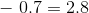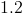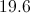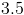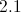Explanation:

To find out what number to subtract 0.7 from to get 2.8, just do the opposite - add 0.7 to 2.8.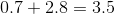### Example Question #1 : Fluently Add, Subtract, Multiply, And Divide Multi Digit Decimals: Ccss.Math.Content.6.Ns.B.3

Find the sum of 2.4, 55, and 6.21.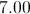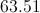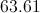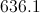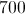Explanation:

To find the sum, you need to add. Line up all numbers vertically. Since one number has a decimal to the tenths place, one has a decimal to the hundredths place, and one has no decimal, be extra careful to line up the digits correctly! To help you, add a zero to the end of 2.4, and add two zeroes to the end of 55 so that all the digits line up correctly.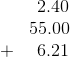Now, add down each column, starting on the right side and moving to the left.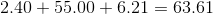The sum is 63.61.

### Example Question #3 : Fluently Add, Subtract, Multiply, And Divide Multi Digit Decimals: Ccss.Math.Content.6.Ns.B.3

Samantha went to the toy store and bought three things:

2 bouncy balls: $0.49 each 1 coloring book:$4.75 each

How much money did Samantha spend at the toy store?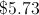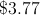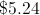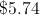Explanation:

To find the answer, you must add up all three items. Be careful to add $0.49 TWO times because Samantha bought 2 bouncy balls: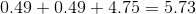Samantha spent$5.73 at the toy store.

### Example Question #1 : Fluently Add, Subtract, Multiply, And Divide Multi Digit Decimals: Ccss.Math.Content.6.Ns.B.3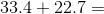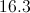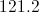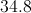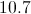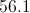Explanation:

Add the "whole" parts of the numbers: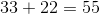Add the "decimal" parts of the numbers: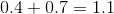Add both parts together: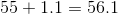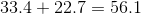### Example Question #5 : Fluently Add, Subtract, Multiply, And Divide Multi Digit Decimals: Ccss.Math.Content.6.Ns.B.3

Solve for: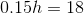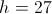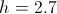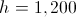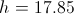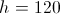Explanation: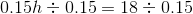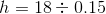To divide, move the decimal point in both numbers right two places to make the divisor whole.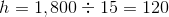### Example Question #6 : Fluently Add, Subtract, Multiply, And Divide Multi Digit Decimals: Ccss.Math.Content.6.Ns.B.3

Find the product.

Steven bought 4 cheeseburgers to share.  Each cheeseburger costs $0.79. How much did Steven pay for the 4 cheeseburgers? Possible Answers: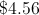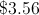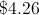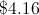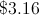Correct answer:Explanation: The easiest way to do this problem is to multiply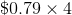. Another option is to add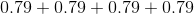. Either way, the correct answer is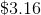. ### Example Question #7 : Fluently Add, Subtract, Multiply, And Divide Multi Digit Decimals: Ccss.Math.Content.6.Ns.B.3 Multiply: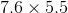Possible Answers: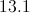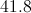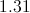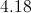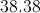Correct answer:Explanation: We ignore the decimals for the time being, multiplying: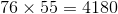Since, between them, the two original factors have a total of two digits to the right of their decimal points, we place the decimal point in the product so that there are two digits to the right. This yields: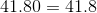### Example Question #1 : Fluently Add, Subtract, Multiply, And Divide Multi Digit Decimals: Ccss.Math.Content.6.Ns.B.3 Gina is recylcing bottles. She earns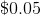for each plastic bottle she recycles and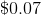cents for each glass bottle she recycles. If Gina recyclesplastic bottles andglass bottles, how much money does she earn? Possible Answers: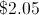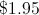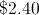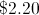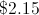Correct answer:Explanation: Multiply the number of plastic bottles Gina recycles by the amount she earns for each plastic bottle, then multiply the number of glass bottles she recycles by the amount she earns for each glass bottle.plastic bottles ateach earns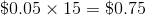glass bottles ateach earns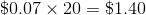Add these earnings together to find Gina's total earnings.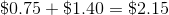### Example Question #1 : Fluently Add, Subtract, Multiply, And Divide Multi Digit Decimals: Ccss.Math.Content.6.Ns.B.3 Dawna wants to buy coffee beans from a local supermarket. If the coffee beans costs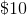a pound, how much would Dawna have to spend on coffee beans if she only wants to buyof a pound? Possible Answers: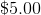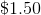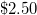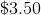Correct answer:Explanation: We have to multiply the cost per pound by the weight that Dawna wants: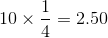This is the same as saying: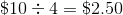. ### Example Question #10 : Fluently Add, Subtract, Multiply, And Divide Multi Digit Decimals: Ccss.Math.Content.6.Ns.B.3 Isaiah buys 5 snacks from the vending machine . If each snack is$1.75, how much money does Isaiah spend?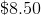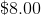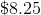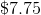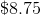Explanation:

Since each snack costs $1.75, multiply$1.75 by 5 to find the total cost: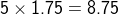← Previous 1 3 4 5 6 7 8 9 22 23

### All Common Core: 6th Grade Math Resources